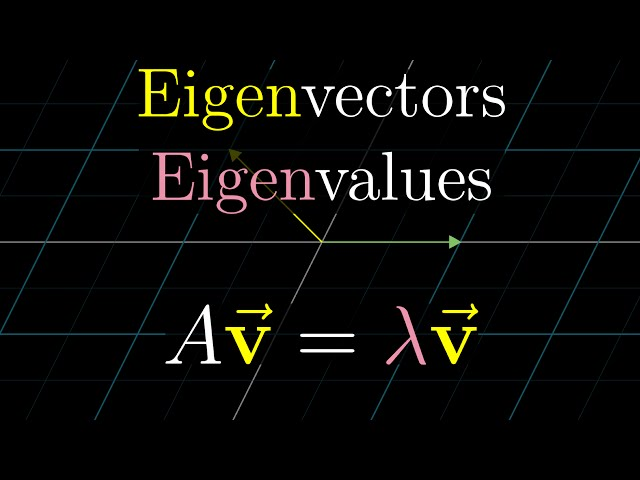# Understanding Eigenvectors and Eigenvalues in Linear AlgebraTLDR Eigenvectors and eigenvalues are useful for understanding linear transformations and can be used to simplify matrix operations.

## Key insights

• 🧠
Understanding eigenvectors and eigenvalues requires a solid visual understanding of matrices as linear transformations, as well as a strong foundation in topics like determinants, linear systems of equations, and change of basis.
• 🧐
Linearity implies that any other vector on a diagonal line spanned by a special vector will also get stretched or squished by a certain factor.
• 💡
Understanding a linear transformation through eigenvectors and eigenvalues provides a deeper insight into what the transformation actually does, regardless of the coordinate system.
• 🧩
The concept of eigenvectors and eigenvalues in linear algebra can be represented symbolically as the matrix-vector product being equal to scaling the eigenvector by a value λ.
• 🎛️
The determinant of a matrix changes as the value of λ changes, and the goal is to find a value of λ that will make the determinant zero, resulting in a transformation that squishes space into a lower dimension.
• 📐
The unaltered matrix has the effect of stretching all the vectors on the diagonal line by a factor of 2.
• 🤔
The concept of an eigenbasis is intriguing as it involves basis vectors that are also eigenvectors, creating a diagonal matrix with eigenvalues on the diagonal and zeros elsewhere.
• 💡
A set of basis vectors that are also eigenvectors is called an "eigenbasis," providing a unique perspective on the transformation and allowing for diagonal representation of the matrix.

## Q&A

• What are eigenvectors and eigenvalues?

Eigenvectors are special vectors that remain on their own line during a transformation, and eigenvalues are the factors by which they are stretched or squished.

• How can eigenvectors and eigenvalues simplify matrix operations?

Eigenvectors and eigenvalues can be used to understand linear transformations and are often a better way to get at the heart of what the transformation does than reading off the columns of the matrix.

• What is the significance of λ in matrix-vector multiplication?

In matrix-vector multiplication, λ represents the corresponding eigenvalue and is used in the transformation associated with the matrix.

• How can diagonal matrices make operations easier?

Diagonal matrices are easier to work with as they can be multiplied by themselves multiple times to scale each basis vector by the corresponding eigenvalue.

• Why is changing to an eigenbasis beneficial?

Changing to an eigenbasis and computing the power of a transformation in that system makes it easier to compute the power in the original coordinate system.

## Timestamped Summary

• 🤔
00:00
Understanding eigenvectors and eigenvalues requires a visual understanding of matrices, determinants, linear systems, and change of basis.
• 🤔
01:26
Matrix stretches x-axis vectors by 3 and diagonal line vectors by 2.
• 🤔
03:04
Eigenvectors and their associated eigenvalues are useful for understanding linear transformations.
• 🤔
05:27
A matrix-vector product of A times v is equal to a scalar-vector multiplication of a matrix times v, with λ as the corresponding eigenvalue, and a matrix-vector multiplication of A minus λ times the identity matrix and a non-zero vector v will always be zero if the transformation associated with the matrix squishes space into a lower dimension.
• 🤔
07:31
Squishing space onto a line with λ=1 gives an eigenvalue of 1.
• 🤔
10:10
Solving for the determinant of a diagonally altered matrix yields the eigenvectors of a 2-D transformation, with the polynomial λ^2+1 having no real number solutions and the shear matrix having only eigenvectors with eigenvalue 1.
• 🔢
12:26
Diagonal matrices make it easier to scale each basis vector by its eigenvalue.
• 🤔
14:28
Find an eigenbasis to simplify matrix operations and uncover unexpected results.Report the articleThank you for the feedback